Courses

# Second Order Process Electrical Engineering (EE) Notes | EduRev

## Electrical Engineering (EE): Second Order Process Electrical Engineering (EE) Notes | EduRev

The document Second Order Process Electrical Engineering (EE) Notes | EduRev is a part of Electrical Engineering (EE) category.
All you need of Electrical Engineering (EE) at this link: Electrical Engineering (EE)

Second Order Process
A second order process is a process whose output is modeled by a second order differential equation.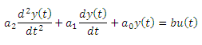66

where, u(t) and y(t) are input and output of the process respectively. If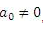, then define the following: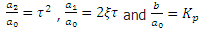Hence, the second order differential equation takes the following form: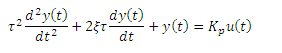67

At steady state condition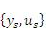, the equation can be re-written as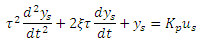68

Subtracting eq. (68) from eq. (67), we obtain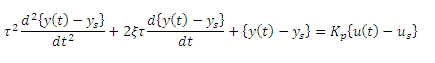69

Alternatively,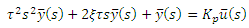70

Where,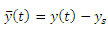and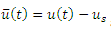are respectively the deviation forms of the output and input of the process around the steady state, whose initial conditions are assumed to be the following: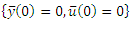Taking Laplace Transform of the eq. (70) we obtain,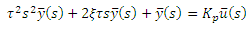71

Rearranging the above we obtain,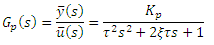72

Kp is called the gain of the process.

Example of a second order process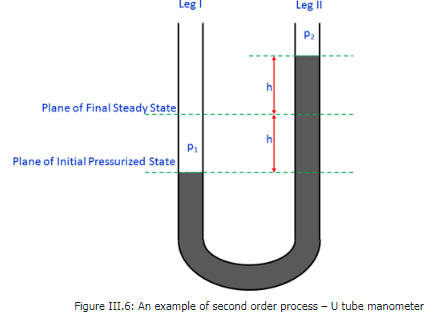Consider the U tube manometer as in Fig.6. The liquid inside the manometer has been shown in a pressurized state. Initially mercury levels at both the legs were at the same height. The present pressurized state is obtained upon exerting a pressure of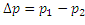on Leg I.

Applying force balance on both the legs of the manometer across plane of initial pressurized state, we obtain: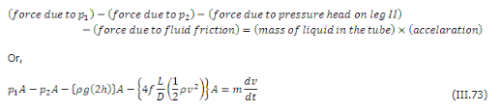Where,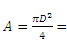cross-sectional area of manometer leg(s), P= density of manometer liquid, f =Fanning' friction factor, v = velocity of manometer liquid, D = diameter of manometer leg(s), L =length of manometer liquid in the tube, m = mass of manometer liquid. Assuming laminar flow inside the manometer, the friction factor can be expressed as ,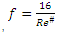where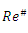is the Reynold's number. Hence the force balance equation takes the form: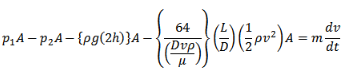74

or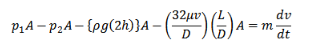75

The velocity of manometer liquid is rate of change of h . Hence,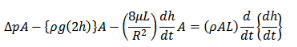76

or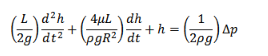77

Comparing eq.(77) with eq.(67), the following can be obtained: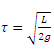and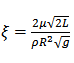and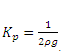.

Dynamic Response of a Second Order Process to a Step Change in the Input

For a step input of magnitude , the Laplace Transform of u(t) would be,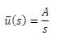78

Hence, second order process takes the following form,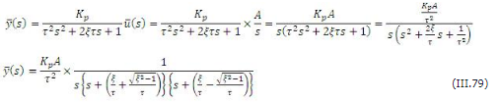The process response will grossly depend upon the value of ξ and there can be three distinguished cases of ξ, i.e. ξ >1; ξ = 1 and ξ <1 .

Case A: ξ = 1

In this case the process response equation in the Laplace domain takes the following form: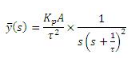80

Using the following: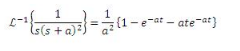81

in eq. (80), we obtain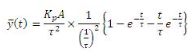82

or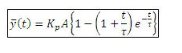83

For ξ ≠ 1, using the following: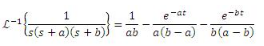84

in eq. (79), we obtain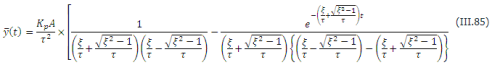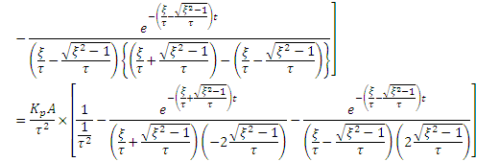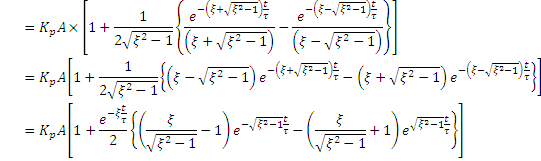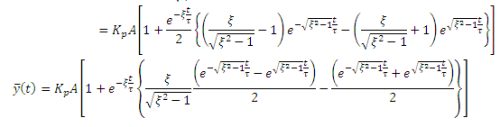Case B: When ξ >1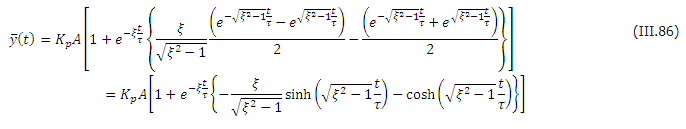In the above equations, the following trigonometric identities have been used: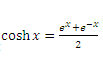andHence we get the final expression for process response when ξ >1,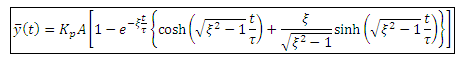87

Case C: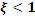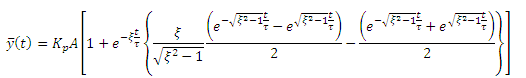88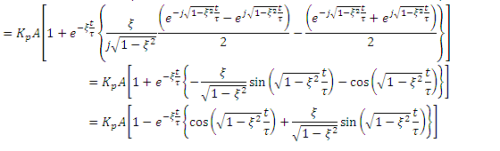In the above equations, the following trigonometric identities have been used: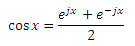89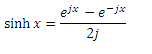90

One can also use the following trigonometric identity for the above expression: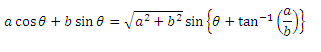91

Hencea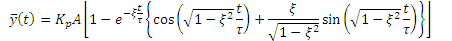92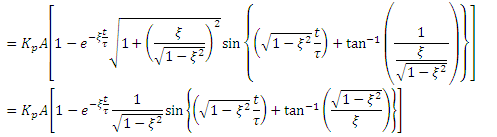Hence we get the final expression for process response for ξ < 1,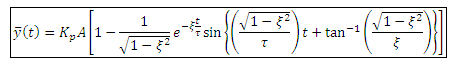93

he frequency of oscillation is94

whereas the phase lag is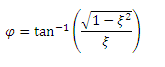95

The document Second Order Process Electrical Engineering (EE) Notes | EduRev is a part of Electrical Engineering (EE) category.
All you need of Electrical Engineering (EE) at this link: Electrical Engineering (EE)Use Code STAYHOME200 and get INR 200 additional OFF Use Coupon Code
All Tests, Videos & Notes of Electrical Engineering (EE): Electrical Engineering (EE)

### Top Courses for Electrical Engineering (EE)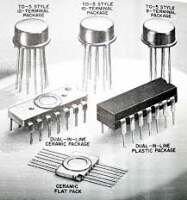### Top Courses for Electrical Engineering (EE)Track your progress, build streaks, highlight & save important lessons and more!

,

,

,

,

,

,

,

,

,

,

,

,

,

,

,

,

,

,

,

,

,

;